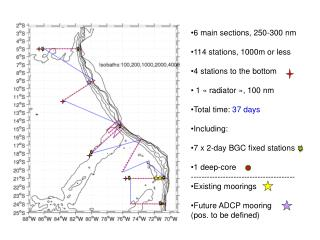DownloadDownload Presentation6 main sections, 250-300 nm 114 stations, 1000m or less 4 stations to the bottom

6 main sections, 250-300 nm 114 stations, 1000m or less 4 stations to the bottom

Download Presentation6 main sections, 250-300 nm 114 stations, 1000m or less 4 stations to the bottom

- - - - - - - - - - - - - - - - - - - - - - - - - - - E N D - - - - - - - - - - - - - - - - - - - - - - - - - - -
Presentation Transcript

1. 6 main sections, 250-300 nm • 114 stations, 1000m or less • 4 stations to the bottom • 1 « radiator », 100 nm • Total time: 37 days • Including: • 7 x 2-day BGC fixed stations • 1 deep-core • --------------------------------------- • Existing moorings • Future ADCP mooring • (pos. to be defined)

2. 4 x 100 nm sections • 30 stations • Time: ~4 days

3. PRIMO cruise parameters (dist in nm, time in days) Section # 1 ; nsta= 15 ; dist = 276.74 ; d_inter_sta= 19.77 ; time (no fix. st.) = 1.93 Transit dist= 451.85 ; transit time= 1.88 (days) Section # 2 ; nsta= 15 ; dist = 276.78 ; d_inter_sta= 19.77 ; time (no fix. st.) = 1.93 Transit dist= 469.46 ; transit time= 1.96 (days) Transit dist= 23.87 ; transit time= 0.10 (days) Section # 3 ; nsta= 8 ; dist = 109.09 ; d_inter_sta= 15.58 ; time (no fix. st.) = 0.87 Transit dist= 136.44 ; transit time= 0.57 (days) Section # 4 ; nsta= 8 ; dist = 114.62 ; d_inter_sta= 16.37 ; time (no fix. st.) = 0.89 Transit dist= 24.68 ; transit time= 0.10 (days) Section # 5 ; nsta= 8 ; dist = 114.85 ; d_inter_sta= 16.41 ; time (no fix. st.) = 0.90 Transit dist= 21.75 ; transit time= 0.09 (days) Section # 6 ; nsta= 15 ; dist = 302.51 ; d_inter_sta= 21.61 ; time (no fix. st.) = 2.04 Transit dist= 372.23 ; transit time= 1.55 (days) Section # 7 ; nsta= 15 ; dist = 267.06 ; d_inter_sta= 19.08 ; time (no fix. st.) = 1.89 Transit dist= 185.39 ; transit time= 0.77 (days) Transit dist= 190.31 ; transit time= 0.79 (days) Section # 8 ; nsta= 15 ; dist = 258.78 ; d_inter_sta= 18.48 ; time (no fix. st.) = 1.86 Transit dist= 180.00 ; transit time= 0.75 (days) Section # 9 ; nsta= 15 ; dist = 234.59 ; d_inter_sta= 16.76 ; time (no fix. st.) = 1.76 Nb_stations (incl. fix. st.)= 114 T_route= 16.71 ; t_sta (incl. 7 fix. and 4 deep st.)= 20.44 (days) T_total (incl. 1 deep core)= 37.40 (days)